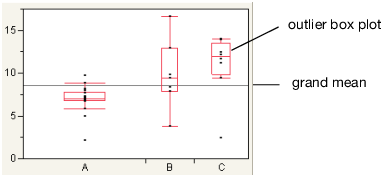Publication date: 11/10/2021

# Quantiles

The Quantiles report lists selected percentiles for each level of the X factor variable. The median is the 50th percentile, and the 25th and 75th percentiles are called the quartiles.

The Quantiles option adds the following elements to the plot:

the grand mean representing the overall mean of the Y variable

outlier box plots summarizing the distribution of points at each factor level

Figure 6.6 Outlier Box Plot and Grand MeanNote: To hide these elements, click the red triangle next to Oneway Analysis and select Display Options > Box Plots or Grand Mean.